# Relationship Between HNM82 Models and T1 Coordinates

## Preamble

In the mid-1990s I invested time trying to gain a better understanding of the "3rd Integral of Motion" that is discussed especially in the context of galaxy dynamics. For example, BT87 discuss the behavior of particle orbits in static potentials in which equipotential contours are nested oblate spheroidal surfaces with uniform eccentricity. This set of equipotential contours does not conform to, and therefore cannot be defined by, the traditional Oblate Spheroidal Coordinate system — detailed, for example, in MF53 — because in that traditional coordinate system surfaces of constant ξ1 are confocal rather than concentric oblate spheroids. In an effort to uncover a closed-form mathematical prescription for the "3rd integral" in this case, I developed an orthogonal coordinate system in which surfaces of constant ξ1 are concentric oblate spheroids. The properties of this T1 coordinate system are detailed in the Appendix of the original version of this H_Book. Here are two relevant links:

I have just realized (in May, 2010) that there is a connection between this T1 Coordinate system and the equipotential contours that arise from at least one of the equilibrium models of the axisymmetric structure of rotationally flattened isothermal gas clouds presented by Hayashi, Narita & Miyama (1982; hereafter HNM82). What follows is a discussion of this connection.

## T1 Coordinates

A spheroidal surface with semi-axes a1 & a3 specified, respectively, along the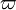$\varpi$ and z axes will be defined by the expression,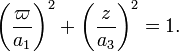$\biggl(\frac{\varpi}{a_1}\biggr)^2 + \biggl(\frac{z}{a_3}\biggr)^2 = 1 .$

Hence, a coordinate system that perfectly overlays a set of concentric spheroidal surfaces should have a radial ξ1 coordinate of the form,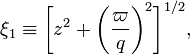$\xi_1 \equiv \biggl[ z^2 + \biggl( \frac{\varpi}{q}\biggr)^2 \biggr]^{1/2} ,$

where the degree of flattening of the concentric surfaces is specified by the (constant) coefficient,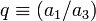$q \equiv (a_1/a_3)$. (The spheroidal surfaces will be oblate if q > 1 and prolate if q < 1.)

A complementary meridional-plane angular coordinate that is everywhere orthogonal to this radial coordinate is,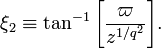$\xi_2 \equiv \tan^{-1}\biggl[ \frac{\varpi}{z^{1/q^2}} \biggr] .$

These are the essential elements of the so-called T1 Coordinate system. Because we are only dealing here with axisymmetric configurations, the third coordinate, which is everywhere orthogonal to the first two, is the familiar azimuthal coordinate,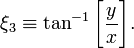$\xi_3 \equiv \tan^{-1}\biggl[ \frac{y}{x} \biggr] .$

### One Special Case

For the specific case of q2 = 2 — that is, for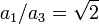$a_1/a_3 = \sqrt{2}$ — we will find that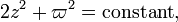$2z^2 + \varpi^2 = \mathrm{constant} ,$

along surfaces of constant ξ1; and we will find that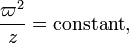$\frac{\varpi^2}{z} = \mathrm{constant} ,$

along surfaces of constant ξ2.

## HNM82

As we have detailed in a chapter entitled,"Rotationally Flattened Isothermal Structures", HNM82 derived a family of equilibrium models of rotationally flattened isothermal gas clouds whose gravitational potential is defined via the analytic expression,

 Φ =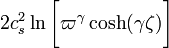$2c_s^2 \ln\biggl[ \varpi^\gamma \cosh(\gamma\zeta) \biggr]$ =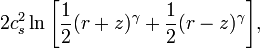$2c_s^2 \ln\biggl[ \frac{1}{2}(r+z)^\gamma + \frac{1}{2}(r-z)^\gamma \biggr] ,$

where γ is an index that identifies the specific member of the family of solutions, cs is the isothermal sound speed, r is a spherical radius, that is,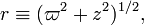$r \equiv ( \varpi^2 + z^2 )^{1/2} ,$

and,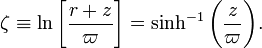$\zeta \equiv \ln\biggl[ \frac{r + z}{\varpi} \biggr] = \sinh^{-1}\biggl(\frac{z}{\varpi}\biggr) .$

For completeness we note that these HNM82 models have a simple rotation profile in which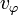$v_\varphi$ is uniform in space, and the index γ is related to the uniform Mach number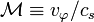$\mathcal{M} \equiv v_\varphi/c_s$ via the algebraic expression,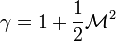$\gamma = 1 + \frac{1}{2}\mathcal{M}^2$ .

Immediately following their Eq. (3.4), HNM82 point out that "lines of gravity force which are perpendicular to the equi-potential surfaces are given by,"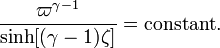$\frac{\varpi^{\gamma-1}}{\sinh[(\gamma-1)\zeta]} = \mathrm{constant} .$ .

### One Special Case

HNM82 also point out that, "in a special case γ = 2, the equi-potential surfaces and the gravity lines are expressed simply as,"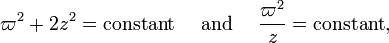$\varpi^2 + 2z^2 = \mathrm{constant} ~~~~\mathrm{and}~~~~ \frac{\varpi^2}{z} = \mathrm{constant} ,$

respectively.

## Relationship Between the Two (to be checked and perhaps extended by Jay Call)

The pair of HNM83 coordinate lines that correspond to the index γ = 2 matches identically the T1 coordinate lines in the special case highlighted above, namely, the case of q2 = 2. At the very least, this must mean that the equilibrium model constructed by HNM82 in the case of γ = 2 must have equipotential surfaces that are concentric oblate spheroids having axis ratio,$a_1/a_3 = \sqrt{2}$.

This makes me wonder whether I ought to redefine the T1 coordinates to conform more to the HNM82 notation. In particular, should I think in terms of hyperbolic rather than trigonometric functions; and should I explore the utility of the coordinate "angle" that HNM82 refer to as ζ?

### T2 Coordinates

#### Trial Definition

If I define the dimensionless angle,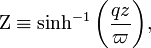$\Zeta \equiv \sinh^{-1}\biggl( \frac{qz}{\varpi} \biggr) ,$

which is similar but not identical to the HNM82 angle, ζ, the two key "T2" coordinates can be written as,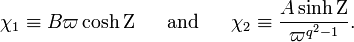$\chi_1 \equiv B \varpi \cosh\Zeta ~~~~~\mathrm{and} ~~~~~ \chi_2 \equiv \frac{A \sinh\Zeta}{ \varpi^{q^2-1}} .$

(To maintain some flexibility, I have inserted two, as yet unspecified, coefficients, B and A, into the expression for χ1 and χ2, respectively.) Let's check to see how these two coordinates are related to the original T1 coordinates: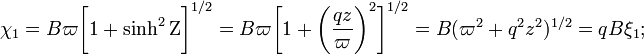$\chi_1 = B \varpi \biggl[ 1+\sinh^2\Zeta \biggr]^{1/2} = B \varpi \biggl[ 1+\biggl( \frac{qz}{\varpi} \biggr)^2 \biggr]^{1/2} = B ( \varpi^2 + q^2z^2 )^{1/2} = qB \xi_1 ;$

and,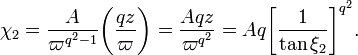$\chi_2 = \frac{A}{ \varpi^{q^2-1}} \biggl( \frac{qz}{\varpi} \biggr) = \frac{Aqz}{\varpi^{q^2}} = Aq \biggl[\frac{1}{\tan\xi_2} \biggr]^{q^2} .$

While the relationship between χ2 and ξ2 looks rather convoluted, there is at least a rational reason (provided by HNM82) for specifying the form of χ2, whereas the original specification of ξ2 was chosen rather arbitrarily in an effort to look like spherical coordinates.

We should probably address as well the structure of the awkward function that appears in the denominator of the T1-coordinate scale factors, namely,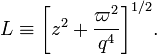$L \equiv \biggl[ z^2 + \frac{\varpi^2}{q^4} \biggr]^{1/2} .$

In terms of our newly introduced hyperbolic functions, this may be written as,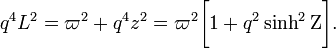$q^4 L^2 = \varpi^2 + q^4 z^2 = \varpi^2 \biggl[1 + q^2 \sinh^2 \Zeta \biggr] .$

#### Scale Factors

To determine the scale factors and position vector, we need to first derive a variety of partial derivatives.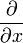$\frac{\partial}{\partial x}$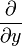$\frac{\partial}{\partial y}$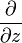$\frac{\partial}{\partial z}$ χ1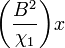$\biggl(\frac{B^2}{\chi_1}\biggr) x$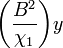$\biggl(\frac{B^2}{\chi_1}\biggr) y$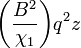$\biggl(\frac{B^2}{\chi_1}\biggr) q^2 z$ χ2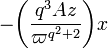$- \biggl( \frac{q^3 A z}{\varpi^{q^2+2}} \biggr) x$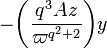$- \biggl( \frac{q^3 A z}{\varpi^{q^2+2}} \biggr) y$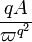$\frac{qA}{\varpi^{q^2}}$ χ3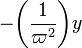$- \biggl( \frac{1}{\varpi^{2}} \biggr) y$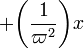$+ \biggl( \frac{1}{\varpi^{2}} \biggr) x$ 0

The scale factors are, therefore: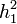$h_1^2$ =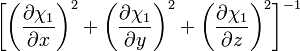$\biggl[ \biggl( \frac{\partial\chi_1}{\partial x} \biggr)^2 + \biggl( \frac{\partial\chi_1}{\partial y} \biggr)^2 + \biggl( \frac{\partial\chi_1}{\partial z} \biggr)^2 \biggr]^{-1}$ =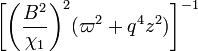$\biggl[ \biggl( \frac{B^2}{\chi_1}\biggr)^2 (\varpi^2 + q^4 z^2) \biggr]^{-1}$ =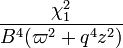$\frac{\chi_1^2}{B^4 (\varpi^2 + q^4 z^2)}$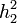$h_2^2$ =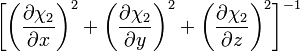$\biggl[ \biggl( \frac{\partial\chi_2}{\partial x} \biggr)^2 + \biggl( \frac{\partial\chi_2}{\partial y} \biggr)^2 + \biggl( \frac{\partial\chi_2}{\partial z} \biggr)^2 \biggr]^{-1}$ =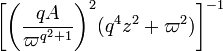$\biggl[ \biggl( \frac{q A}{\varpi^{q^2+1}}\biggr)^2 (q^4 z^2 + \varpi^2) \biggr]^{-1}$ =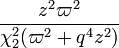$\frac{z^2 \varpi^2 }{\chi_2^2 (\varpi^2 + q^4 z^2)}$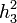$h_3^2$ =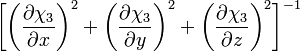$\biggl[ \biggl( \frac{\partial\chi_3}{\partial x} \biggr)^2 + \biggl( \frac{\partial\chi_3}{\partial y} \biggr)^2 + \biggl( \frac{\partial\chi_3}{\partial z} \biggr)^2 \biggr]^{-1}$ =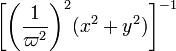$\biggl[ \biggl( \frac{1}{\varpi^2}\biggr)^2 (x^2 + y^2) \biggr]^{-1}$ =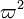$\varpi^2$

#### Position Vector

When written in terms of the T2-coordinate unit vectors, the Cartesian unit vectors are:$\hat{i}$ =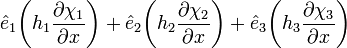$\hat{e}_1 \biggl( h_1 \frac{\partial\chi_1}{\partial x} \biggr) + \hat{e}_2 \biggl( h_2 \frac{\partial\chi_2}{\partial x} \biggr) + \hat{e}_3 \biggl( h_3 \frac{\partial\chi_3}{\partial x} \biggr)$ =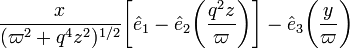$\frac{x}{(\varpi^2 + q^4 z^2)^{1/2}} \biggl[ \hat{e}_1 - \hat{e}_2\biggl(\frac{q^2 z}{\varpi}\biggr) \biggr] - \hat{e}_3 \biggl(\frac{y}{\varpi}\biggr)$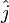$\hat{j}$ =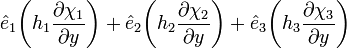$\hat{e}_1 \biggl( h_1 \frac{\partial\chi_1}{\partial y} \biggr) + \hat{e}_2 \biggl( h_2 \frac{\partial\chi_2}{\partial y} \biggr) + \hat{e}_3 \biggl( h_3 \frac{\partial\chi_3}{\partial y} \biggr)$ =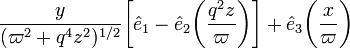$\frac{y}{(\varpi^2 + q^4 z^2)^{1/2}} \biggl[ \hat{e}_1 - \hat{e}_2\biggl(\frac{q^2 z}{\varpi}\biggr) \biggr] + \hat{e}_3 \biggl(\frac{x}{\varpi}\biggr)$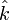$\hat{k}$ =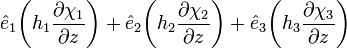$\hat{e}_1 \biggl( h_1 \frac{\partial\chi_1}{\partial z} \biggr) + \hat{e}_2 \biggl( h_2 \frac{\partial\chi_2}{\partial z} \biggr) + \hat{e}_3 \biggl( h_3 \frac{\partial\chi_3}{\partial z} \biggr)$ =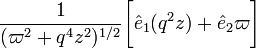$\frac{1}{(\varpi^2 + q^4 z^2)^{1/2}} \biggl[ \hat{e}_1 (q^2 z) + \hat{e}_2 \varpi \biggr]$

Therefore, the position vector is,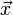$\vec{x}$ =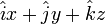$\hat{i}x + \hat{j}y + \hat{k}z$ =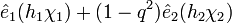$\hat{e}_1 (h_1 \chi_1) + (1 - q^2) \hat{e}_2 (h_2 \chi_2)$

### Coordinate Inversion

Can we invert these expressions to obtain$\varpi$ and Ζ (or, equivalently, z) strictly in terms of χ1 and χ2? Well, we can start by squaring both coordinates and subtracting to isolate$\varpi^2$. Specifically,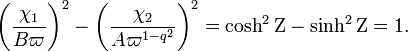$\biggl( \frac{\chi_1}{B\varpi} \biggr)^2 - \biggl( \frac{\chi_2}{A\varpi^{1-q^2}} \biggr)^2 = \cosh^2\Zeta - \sinh^2\Zeta = 1 .$

Hence, the desired functional expression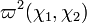$\varpi^2(\chi_1,\chi_2)$ comes from the root(s) of the following polynomial equation: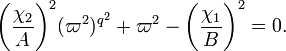$\biggl( \frac{\chi_2}{A} \biggr)^2 (\varpi^2)^{q^2} + \varpi^2 - \biggl(\frac{\chi_1}{B}\biggr)^2 = 0 .$

It seems unlikely that closed-form analytic expressions can be extracted for the root(s) of this equation for an arbitrary choice of the flattening index, q. But it should be possible to obtain analytically expressible roots in the case of q2 = 2, q2 = 3, and q2 = 4. Once the expression for$\varpi^2(\chi_1,\chi_2)$ is known, a determination of z12) is straightforward. Specifically,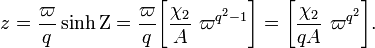$z = \frac{\varpi}{q} \sinh\Zeta = \frac{\varpi}{q} \biggl[ \frac{\chi_2}{A} ~ \varpi^{q^2-1} \biggr] = \biggl[ \frac{\chi_2}{qA} ~ \varpi^{q^2} \biggr] .$

In addition, from the above polynomial expression, we know that,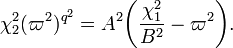$\chi_2^2 (\varpi^2)^{q^2} = A^2 \biggl(\frac{\chi_1^2}{B^2} - \varpi^2 \biggr) .$

Hence, quite generally we deduce,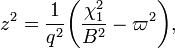$z^2 = \frac{1}{q^2} \biggl(\frac{\chi_1^2}{B^2} - \varpi^2 \biggr) ,$

which, in retrospect, could have been derived more directly from the definition of χ1.

When q2 = 2, the above polynomial becomes a quadratic equation and therefore its roots can be determined analytically. Setting,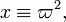$x \equiv \varpi^2 ,$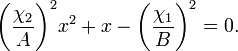$\biggl( \frac{\chi_2}{A} \biggr)^2 x^2 + x - \biggl( \frac{\chi_1}{B} \biggr)^2 = 0 .$

The root of this function that provides positive values for x (i.e., for$\varpi^2$) is,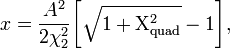$x = \frac{A^2}{2\chi_2^2} \biggl[ \sqrt{1 + \Chi_\mathrm{quad}^2} - 1 \biggr] ,$

where,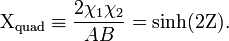$\Chi_\mathrm{quad} \equiv \frac{2\chi_1 \chi_2}{AB} = \sinh (2\Zeta).$

This is the desired inversion which gives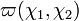$\varpi(\chi_1,\chi_2)$ in the case q2 = 2. The desired expression for z12) is,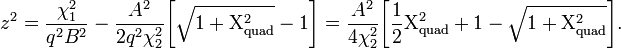$z^2 = \frac{\chi_1^2}{q^2 B^2}- \frac{A^2}{2q^2 \chi_2^2} \biggl[ \sqrt{1 + \Chi_\mathrm{quad}^2} - 1 \biggr] = \frac{A^2}{4 \chi_2^2}\biggl[ \frac{1}{2} \Chi_\mathrm{quad}^2 +1 - \sqrt{1 + \Chi_\mathrm{quad}^2}\biggr] .$

We note as well that in this special case, where q2 = 2,

 4L2 =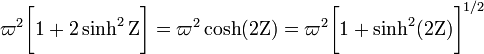$\varpi^2 \biggl[1 + 2 \sinh^2 \Zeta \biggr] = \varpi^2 \cosh(2\Zeta) = \varpi^2 \biggl[ 1+\sinh^2(2\Zeta) \biggr]^{1/2}$ =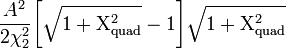$\frac{A^2}{2\chi_2^2} \biggl[ \sqrt{1 + \Chi_\mathrm{quad}^2} - 1 \biggr] \sqrt{ 1 + \Chi_\mathrm{quad}^2}$ =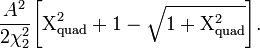$\frac{A^2}{2\chi_2^2} \biggl[ \Chi_\mathrm{quad}^2 + 1 - \sqrt{ 1 + \Chi_\mathrm{quad}^2} \biggr] .$

Note that this expression is very similar, but not identical, to the expression just derived for z2.

### Second Special Case (cubic)

When q2 = 3, the above polynomial becomes a cubic equation and therefore its roots also can be determined analytically. Again setting,$x \equiv \varpi^2 ,$

the relevant cubic equation is,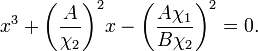$x^3 + \biggl( \frac{A}{\chi_2} \biggr)^2 x - \biggl( \frac{A \chi_1}{B \chi_2} \biggr)^2 = 0 .$

A cubic equation of the form,

x3 + ax + b = 0,

has the following real root:

x = α + β,

where,

 α3 =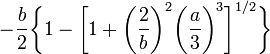$-\frac{b}{2} \biggl\{ 1 - \biggl[ 1 + \biggl( \frac{2}{b} \biggr)^2 \biggl( \frac{a}{3} \biggr)^3 \biggr]^{1/2} \biggr\}$ β3 =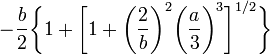$-\frac{b}{2} \biggl\{ 1 + \biggl[ 1 + \biggl( \frac{2}{b} \biggr)^2 \biggl( \frac{a}{3} \biggr)^3 \biggr]^{1/2} \biggr\}$

The root of this function that provides positive values for x (i.e., for$\varpi^2$) is,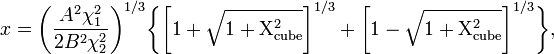$x = \biggl( \frac{A^2 \chi_1^2}{2 B^2\chi_2^2} \biggr)^{1/3} \biggl\{ \biggl[ 1 + \sqrt{1 + \Chi_\mathrm{cube}^2} \biggr]^{1/3} + \biggl[ 1 - \sqrt{1 + \Chi_\mathrm{cube}^2} \biggr]^{1/3} \biggr\} ,$

where,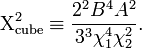$\Chi_\mathrm{cube}^2 \equiv \frac{2^2 B^4 A^2}{3^3 \chi_1^4 \chi_2^2} .$

This is the desired inversion which gives$\varpi(\chi_1,\chi_2)$ in the case q2 = 3.

## Integrals of Motion

T2 Coordinates: In an accompanying chapter we begin to examine what the 3rd integral of motion might be in the T2 coordinate system.

T3 Coordinates: In yet another accompanying chapter we define the properties of, and begin to examine what the 3rd integral of motion might be, in a system we refer to as T3 coordinates.